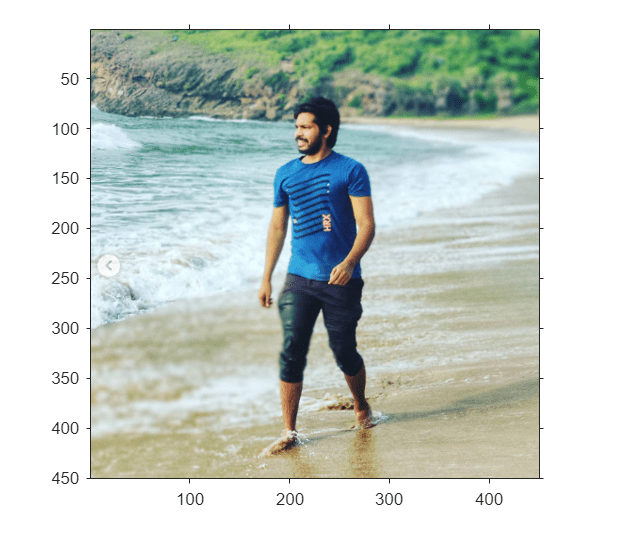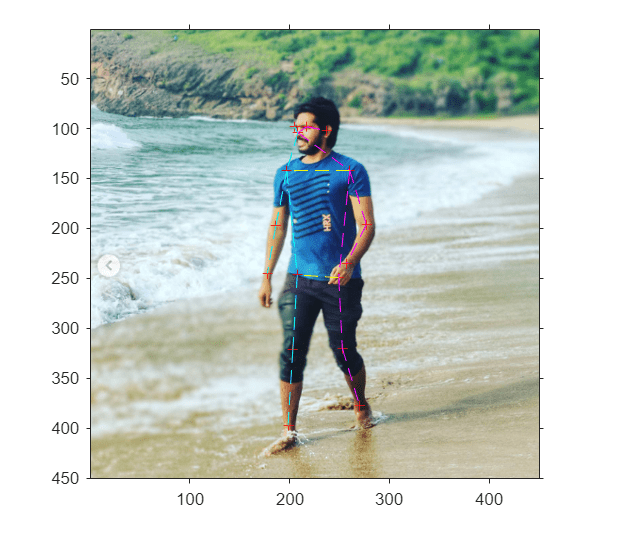# Deploy Pose Estimation Application Using TensorFlow Lite Model (TFLite) Model on Host and Raspberry Pi

This example shows simulation and code generation of a TensorFlow Lite model for 2D human pose estimation.

Human pose estimation is the task of predicting the pose of a human subject in an image or a video frame by estimating the spatial locations of joints such as elbows, knees, or wrists (keypoints). This example uses the MoveNet TensorFlow Lite pose estimation model from TensorFlow hub.

The pose estimation models takes a processed camera image as the input and outputs information about keypoints. The keypoints detected are indexed by a part ID, with a confidence score between 0.0 and 1.0. The confidence score indicates the probability that a keypoint exists in that position. This example can be used to estimate 17 keypoints.

### Third-Party Prerequisites

This example uses MoveNet; a state-of-the-art pose estimation model. Download the model file from the TFLite website. The model file is approximately 9.4 MB in size.

```if ~exist("Movenet.tflite","file") disp('Downloading MoveNet lightning model file...'); url = "https://tfhub.dev/google/lite-model/movenet/singlepose/lightning/" + ... "3?lite-format=tflite"; websave("Movenet.tflite",url); end```
```Downloading MoveNet lightning model file... ```

To load the TensorFlow Lite model, use the `loadTFLiteModel` function. The properties of the `TFLiteModel` object contains information such as the size and number of inputs and outputs in the model. For more information, see `loadTFLiteModel` and `TFLiteModel`.

`net = loadTFLiteModel('Movenet.tflite');`

Inspect the T`FLiteModel` object.

`disp(net)`
``` TFLiteModel with properties: ModelName: 'Movenet.tflite' NumInputs: 1 NumOutputs: 1 InputSize: {[192 192 3]} OutputSize: {[1 17 3]} InputScale: 1 InputZeroPoint: 0 OutputScale: 1 OutputZeroPoint: 0 InputType: {["SINGLE"]} OutputType: {["SINGLE"]} NumThreads: 6 Mean: 127.5000 StandardDeviation: 127.5000 ```

The input to the model is a frame of video or an image of size 192-by-192-by-3. The output is of size 1-by-17-by-3. The first two channels of the last dimension is the yx coordinates of the 17 keypoints. The third channel of the last dimension represents the accuracy of the prediction for each keypoint.

Use `importdata` to load keypoints data. A keypoints data encapsulates the keypoints of human body.

`labelsFile = importdata('keypoints.txt');`

Display the keypoints.

`disp(labelsFile)`
``` {'nose' } {'left_eye' } {'right_eye' } {'left_ear' } {'right_ear' } {'left_shoulder' } {'right_shoulder'} {'left_elbow' } {'right_elbow' } {'left_wrist' } {'right_wrist' } {'left_hip' } {'right_hip' } {'left_knee' } {'right_knee' } {'left_ankle' } {'right_ankle' } ```

### Display the test image

Read image on which pose estimation has to be done.

```I = imread('poseEstimationTestImage.png'); I1 = imresize(I,[192 192]); % Pose estimation will be displayed on High Resolution Image I2 = imresize(I,[450 450]); imshow(I2); axis on; hold on;```### The tflite_pose_estimation_predict Entry-Point Function

The `tflite_pose_estimation_predict` entry-point function loads the MoveNet model into a persistent `TFLiteModel` object by using the `loadTFLiteModel` function.

`type tflite_pose_estimation_predict.m`
```function out = tflite_pose_estimation_predict(in) % Copyright 2022-2023 The MathWorks, Inc. persistent net; if isempty(net) net = loadTFLiteModel('Movenet.tflite'); % Set Number of Threads based on number of threads in hardware % net.NumThreads = 4; end net.Mean = 0; net.StandardDeviation = 1; out = net.predict(in); end ```

### Run MATLAB Simulation on Host

Run the simulation by passing the input image `I1` to the entry-point function. This output is further processed in post processing block.

`output = tflite_pose_estimation_predict(I1);`

#### Plot the Keypoints of the Image

Set threshold Value while drawing lines to connect keypoints.

`threshold = 0.29;`

Get the X and Y coordinates of the keypoints. In output, First channel represents Y coordinates, Second channel represents X coordinates, Third channel represents accuracy of the keypoints.

```[KeyPointXi,KeyPointYi,KeyPointAccuracy] = getKeyPointValues(net,output, ... size(I2));```

Plot the keypoints which are more than threshold value. Calling `PlotKeyPoints` without the `threshold` argument will plot all the keypoints of the image.

`PlotKeyPointsImage(net,KeyPointXi,KeyPointYi,KeyPointAccuracy,threshold);`

Connect keypoints of the Image. Calling `ConnectKeyPoints` without the `threshold` argument will connect all keypoints

`ConnectKeyPointsImage(KeyPointXi,KeyPointYi,KeyPointAccuracy,threshold);`### Generate MEX Function for Pose Estimation

To generate a MEX function for a specified entry-point function, create a code configuration object for a MEX. Set the target language to C++.

```cfg = coder.config('mex'); cfg.TargetLang = 'C++';```

Run the `codegen` command to generate the MEX function `tflite_pose_estimation_predict_mex` on the host platform.

`codegen -config cfg tflite_pose_estimation_predict -args ones(192,192,3,'single')`
```Code generation successful. ```

#### Run Generated MEX

Run the generated MEX by passing the input image `I1`. This output is further processed in post processing block.

`output = tflite_pose_estimation_predict_mex(single(I1));`

#### Plot the Keypoints of the Image

```threshold = 0.29; [KeyPointXi,KeyPointYi,KeyPointAccuracy] = getKeyPointValues(net,output, ... size(I2)); PlotKeyPointsImage(net,KeyPointXi,KeyPointYi,KeyPointAccuracy,threshold); ConnectKeyPointsImage(KeyPointXi,KeyPointYi,KeyPointAccuracy,threshold);```### Deploy Pose Estimation Application to Raspberry Pi

#### Set Up Connection with Raspberry Pi

Use the MATLAB Support Package for Raspberry Pi Hardware function `raspi` to create a connection to the Raspberry Pi.

The `raspi` function reuses these settings from the most recent successful connection to the Raspberry Pi hardware. This example establishes an SSH connection to the Raspberry Pi hardware using the settings stored in memory.

`r = raspi;`

If this is the first time connecting to a Raspberry Pi board or if you want to connect to a different board, use the following line of code:

```r = raspi('raspiname','username','password'); ```

#### Copy TFLite Model to Target Hardware

Copy TFLite Model to the Raspberry Pi board. On the hardware board, set the environment variable `TFLITE_MODEL_PATH` to the location of the TFLite model. For more information on setting environment variables, see Prerequisites for Deep Learning with TensorFlow Lite Models.

In the following commands, replace `targetDir` with the destination folder of TFLite model on the Raspberry Pi board.

`r.putFile('Movenet.tflite','/home/pi');`

#### Generate PIL MEX Function

To generate a PIL MEX function for a specified entry-point function, create a code configuration object for a static library and set the verification mode to `'PIL'`. Set the target language to C++.

```cfg = coder.config('lib','ecoder',true); cfg.TargetLang = 'C++'; cfg.VerificationMode = 'PIL';```

Create a `coder.hardware` (MATLAB Coder) object for Raspberry Pi and attach it to the code generation configuration object.

```hw = coder.hardware('Raspberry Pi'); cfg.Hardware = hw;```

Run the `codegen` command to generate a PIL MEX function `tflite_pose_estimation_predict_pil`.

`codegen -config cfg tflite_pose_estimation_predict -args ones(192,192,3,'single')`
```### Connectivity configuration for function 'tflite_pose_estimation_predict': 'Raspberry Pi' Location of the generated elf : /home/pi/MATLAB_ws/R2023a/home/lnarasim/Documents/MATLAB/ExampleManager/lnarasim.BR2023ad.j2182215/deeplearning_shared-ex29026693/codegen/lib/tflite_pose_estimation_predict/pil Code generation successful. ```

#### Run Generated PIL

Run the generated MEX by passing the input image `I1`. This output is further processed in post processing block.

`output = tflite_pose_estimation_predict_pil(single(I1));`
```### Starting application: 'codegen/lib/tflite_pose_estimation_predict/pil/tflite_pose_estimation_predict.elf' To terminate execution: clear tflite_pose_estimation_predict_pil ### Launching application tflite_pose_estimation_predict.elf... ```

#### Plot the Keypoints of the Image

```threshold = 0.29; [KeyPointXi,KeyPointYi,KeyPointAccuracy] = getKeyPointValues(net,output, ... size(I2)); PlotKeyPointsImage(net,KeyPointXi,KeyPointYi,KeyPointAccuracy,threshold); ConnectKeyPointsImage(KeyPointXi,KeyPointYi,KeyPointAccuracy,threshold);```